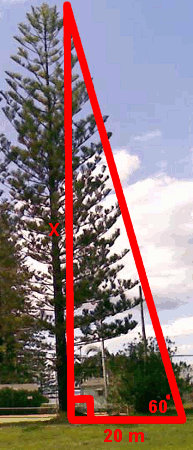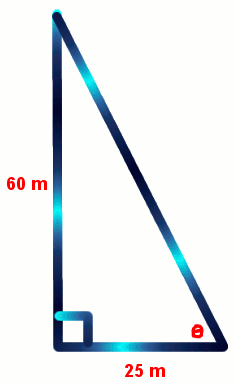# Trigonometry Tangent Rule

There are 3 trigonometry rules which are:

• Sin θ = O/H
• Cos θ = A/H
• Tan θ = O/A (when there is no hypotenuse)

## Example One - Tangent Rule to Find the Opposite Side

A very tall Norfolk Island pine tree is in a park next to my house. Because of its age, it has root rot and is likely to fall on my house if we get a strong wind. I need to find the height of the tree to prove to the local council that it is dangerous and must be chopped down.If I stand 20 metres away from the base of the tree, the angle from my feet to the top of the tree is 60°. What is the height of the tree?

tan θ = O/A
(Always draw a diagram and write the rule. Then substitute the numbers and letters specific to this question. See the next line of working.)

tan 60 = x/20
(If x is on the top of the fraction, multiply both sides of the equation by the number on the bottom which is 20.)

tan 60 × 20 = x
(Now type tan 30 × 20 on your calculator. You may need to close the bracket after the 30.)

x = 34.64

The height of the tree is 34.64 metres.

## Question - Finding the Opposite Side

If I stand 30 metres away from the base of a building, the angle from my feet to the top of the building is 80°. How high is the building? (Draw a diagram.)

170.14 metres

## Example Two - Tangent Rule to Find the Adjacent Side

At a cinema, a wheelchair ramp must be built next to steps that are 3 metres high. For safety, the maximum angle of the ramp is . What must be the horizontal length of the ramp?tan θ = O/A
(Always draw a diagram and write the rule. Then substitute the numbers and letters specific to this question. See the next line of working.)

tan 5 = 3/x
(If x is on the bottom of the fraction, multiply both side by x and divide both sides by tan 5. In effect, you will swap the tan and the side. See the next line of working.)

x = 3 / tan 5
(Now type 3 / tan 5 on your calculator. You may need to close the bracket after the 5.)

x = 34.29

The horizontal length of the ramp must be 34.29 metres.

## Question - Finding the Adjacent Side

A rescue helicopter is flying at an altitude of 2000 metres and is returning to the base. At the base, the waiting paramedics can see that the helicopter is at an angle of 10° from them. What is the horizontal distance that the helicopter is away from the base? (Draw a diagram.)

11342.56 metres

## Example Three - Tangent Rule to Find an Angle

One section of a rollercoaster makes a right-angled triangle shape with a vertical length of 60 metres and a horizontal height of 25 metres. What is the angle that the rollercoaster makes with the ground?tan θ = O/A
(Always draw a diagram and write the rule. Then substitute the numbers and letters specific to this question. See the next line of working.)

tan θ = 60/25

θ = tan-1 (60/25)
(Now type Shift Tan (60/25) on your calculator. Use brackets for the fraction part.)

θ = 67.38°

The angle of the rollercoaster is 67.38°.

## Question - Finding an Angle

Another section of the rollercoaster has a vertical height of 100 metres and a horizontal distance of 50 metres. What angle does it make with the ground?
(Draw a diagram.)